# Make Enriched Heatmaps

Author: Zuguang Gu ( z.gu@dkfz.de )

Date: 2022-04-26

Enriched heatmap is a special type of heatmap which visualizes the enrichment of genomic signals over specific target regions. It is broadly used to visualize e.g. how histone modifications are enriched at transcription start sites.

There are already several tools that are able to make such heatmap (e.g. ngs.plot, deepTools or genomation). Here we implement enriched heatmap by ComplexHeatmap package. Since the enriched heatmap is essentially a normal heatmap but with some special settings, with the functionality of ComplexHeatmap, it would be much easier to customize the heatmaps as well as concatenating to a list of heatmaps to show correspondance between different data sources.

## Basic usages

library(EnrichedHeatmap)


First let's load the example dataset that we will use for demonstration. The data for human lung tissue is from Roadmap dataset.

set.seed(123)
ls()

##  "H3K4me3" "cgi"     "genes"   "meth"    "rpkm"


There are following R objects:

• H3K4me3: coverage for H3K4me3 histone modification from the ChIP-seq data,
• cgi: CpG islands,
• genes: genes,
• meth: methylation for CpG sites from WGBS,
• rpkm: gene expression from RNASeq.

In order to build the vignette fast, the data only includes chromosome 21. Also we downsampled 100000 CpG sites for methylation data.

We first visualize how H3K4me3 histone modification is enriched around gene TSS. We extract TSS of genes (note tss has strand information):

tss = promoters(genes, upstream = 0, downstream = 1)
tss[1:5]

## GRanges object with 5 ranges and 0 metadata columns:
##                      seqnames    ranges strand
##                         <Rle> <IRanges>  <Rle>
##    ENSG00000141956.9    chr21  43299591      -
##   ENSG00000141959.12    chr21  45719934      +
##    ENSG00000142149.4    chr21  33245628      +
##   ENSG00000142156.10    chr21  47401651      +
##    ENSG00000142166.8    chr21  34696734      +
##   -------
##   seqinfo: 1 sequence from an unspecified genome; no seqlengths

H3K4me3[1:5]

## GRanges object with 5 ranges and 1 metadata column:
##       seqnames          ranges strand |  coverage
##          <Rle>       <IRanges>  <Rle> | <integer>
##       chr21 9825468-9825470      * |        10
##       chr21 9825471-9825488      * |        13
##       chr21         9825489      * |        12
##       chr21         9825490      * |        13
##       chr21 9825491-9825493      * |        14
##   -------
##   seqinfo: 1 sequence from an unspecified genome; no seqlengths


Similar as other tools, the task of visualization are separated into two steps:

1. get association between genomic signals and targets by normalizing into a matrix.
2. visualize the matrix by heatmap.
mat1 = normalizeToMatrix(H3K4me3, tss, value_column = "coverage",
extend = 5000, mean_mode = "w0", w = 50)
mat1

## Normalize H3K4me3 to tss:
##   Upstream 5000 bp (100 windows)
##   Downstream 5000 bp (100 windows)
##   Include target regions (width = 1)
##   720 target regions

class(mat1)

##  "normalizedMatrix" "matrix"


normalizeToMatrix() converts the association between genomic signals (H3K4me3) and targets(tss) into a matrix (actually mat1 is just a normal matrix with several additional attributes). It first splits the extended targets regions (the extension to upstream and downstream is controlled by extend argument) into a list of small windows (the width of the windows is controlled by w), then overlaps genomic signals to these small windows and calculates the value for every small window which is the mean value of genomic signals that intersects with the window (the value corresponds to genomic signals are controlled by value_column and how to calcualte the mean value is controlled by mean_mode.).

There are several modes for mean_mode according to different types of genomic signals. It will be explained in later sections.

With mat1, we can visualize it as a heatmap:

EnrichedHeatmap(mat1, name = "H3K4me3")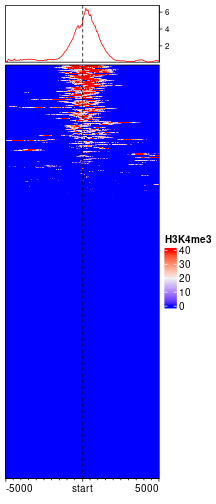By default, rows are ordered according to the enrichment to the target regions. On top of the heatmap there is a specific type of annotation which summarize the enrichment patterns as a line plot, implemented b anno_enriched().

EnrichedHeatmap() internally calls Heatmap() and returns a Heatmap class object, so parameters for Heatmap() can be directly applied to EnrichedHeatmap(). Users can go to the ComplexHeatmap package to get a more comprehensive help.

### Colors

Similar as the normal heatmap, the simplest way to set colors is to provide a vector of colors.

EnrichedHeatmap(mat1, col = c("white", "red"), name = "H3K4me3")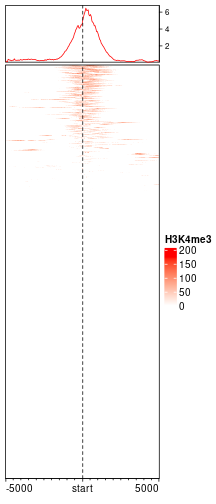You may wonder why the color looks so light. The reason is in coverage values in H3K4me3, there exist some extreme values, which results in extreme value in mat1.

quantile(H3K4me3$coverage, c(0, 0.25, 0.5, 0.75, 0.99, 1))  ## 0% 25% 50% 75% 99% 100% ## 10 18 29 43 87 293  quantile(mat1, c(0, 0.25, 0.5, 0.75, 0.99, 1))  ## 0% 25% 50% 75% 99% 100% ## 0.00000 0.00000 0.00000 0.00000 38.92176 174.78000  If a vector of colors is specified, sequential values from minimal to maximal are mapped to the colors, and other values are linearly interpolated. To get rid of such extreme values, there are two ways. The first is to specify keep option which trims extreme values both at lower and upper bounds. (In following, it means only to trim values larger than 99th percentile.) mat1_trim = normalizeToMatrix(H3K4me3, tss, value_column = "coverage", extend = 5000, mean_mode = "w0", w = 50, keep = c(0, 0.99)) EnrichedHeatmap(mat1_trim, col = c("white", "red"), name = "H3K4me3")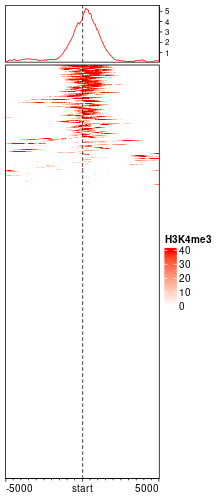The second way is to define a color mapping function which only maps colors to values less than 99th percentile and the value larger than the 99th percentile uses same color as the 99th percentile. library(circlize) col_fun = colorRamp2(quantile(mat1, c(0, 0.99)), c("white", "red")) EnrichedHeatmap(mat1, col = col_fun, name = "H3K4me3")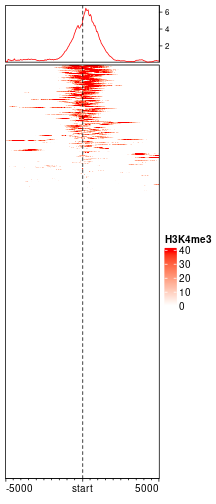To sum it up, the first way directly modified values in mat1 while the second way keeps the original values but uses a robust color mapping. If col is not specified in EnrichedHeatmap(), blue-white-red is mapped to 1st quantile, mean and 99th quantile by default. In following sections, we will also use the matrix to do row-clustering, thus we directly use the trimmed matrix. mat1 = mat1_trim  ### Split on rows Split rows by a vector or a data frame by specifying row_split option. EnrichedHeatmap(mat1, col = col_fun, name = "H3K4me3", row_split = sample(c("A", "B"), length(genes), replace = TRUE), column_title = "Enrichment of H3K4me3")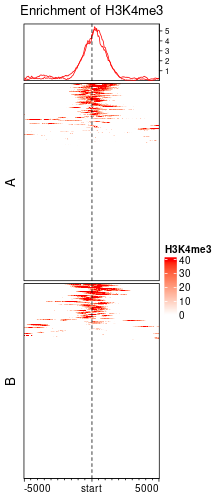Split rows by k-means clustering by specifying row_km option. set.seed(123) EnrichedHeatmap(mat1, col = col_fun, name = "H3K4me3", row_km = 3, column_title = "Enrichment of H3K4me3", row_title_rot = 0)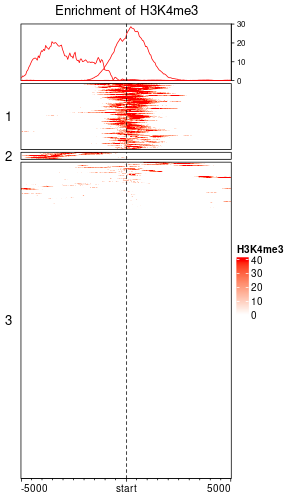In each row cluster, rows are still ordered by the enrichment. When rows are split, graphic parameters for the enriched annotation can be a vector with length as the number of row clusters. set.seed(123) EnrichedHeatmap(mat1, col = col_fun, name = "H3K4me3", row_km = 3, top_annotation = HeatmapAnnotation(enriched = anno_enriched(gp = gpar(col = 2:4, lty = 1:3))), column_title = "Enrichment of H3K4me3", row_title_rot = 0)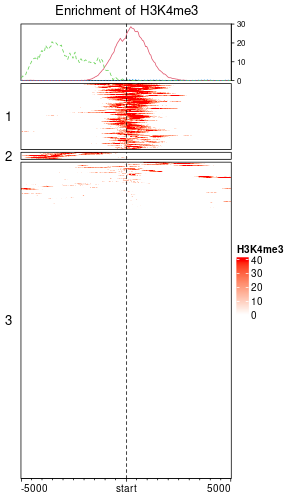Users can go to https://jokergoo.github.io/ComplexHeatmap-reference/book/a-single-heatmap.html#heatmap-split for more controls of splitting heatmaps. ### Cluster on rows Cluster on rows. By default show_row_dend is turned off, so you don't need to specify it here. More options for row clustering can be found in the help page of Heatmap() or https://jokergoo.github.io/ComplexHeatmap-reference/book/a-single-heatmap.html#clustering. EnrichedHeatmap(mat1, col = col_fun, name = "H3K4me3", cluster_rows = TRUE, column_title = "Enrichment of H3K4me3")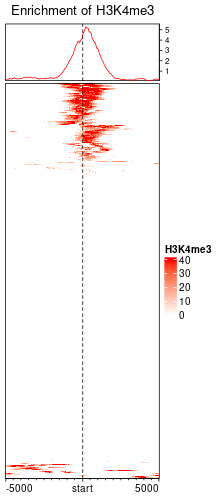Vignette row_ordering.html compares different row ordering methods and clustering methods, and discusses which might be the proper way to show the enrichment patterns. ### Extensions to target regions Extension to upstream and downstream can be controled by extend either by a single value or a vector of length 2. # upstream 1kb, downstream 2kb mat12 = normalizeToMatrix(H3K4me3, tss, value_column = "coverage", extend = c(1000, 2000), mean_mode = "w0", w = 50) EnrichedHeatmap(mat12, name = "H3K4me3", col = col_fun)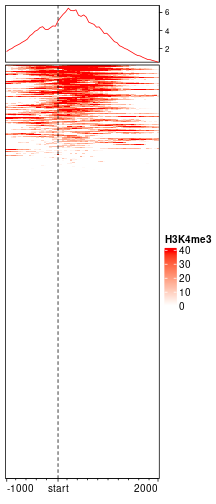Either upstream or downstream can be set to 0. mat12 = normalizeToMatrix(H3K4me3, tss, value_column = "coverage", extend = c(0, 2000), mean_mode = "w0", w = 50) EnrichedHeatmap(mat12, name = "H3K4me3", col = col_fun)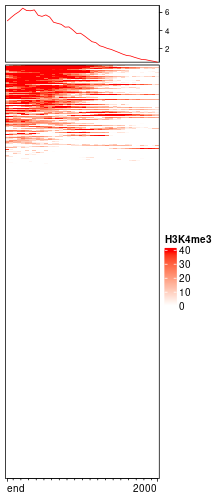mat12 = normalizeToMatrix(H3K4me3, tss, value_column = "coverage", extend = c(1000, 0), mean_mode = "w0", w = 50) EnrichedHeatmap(mat12, name = "H3K4me3", col = col_fun)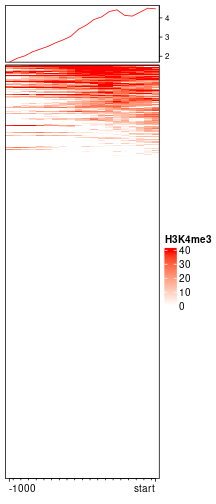Sometimes whether the signals are on the upstream or the downstream of the targets are not important and users only want to show the relative distance to targets. If flip_upstream is set to TRUE, the upstream part in the normalized matrix is flipped and added to the downstream part. The flipping is only allowed when the targets are single-point targets or the targets are excluded in the normalized matrix (by setting include_target = FALSE). If the extension for the upstream and downstream is not equal, the smaller extension is used for the final matrix. mat_f = normalizeToMatrix(H3K4me3, tss, value_column = "coverage", extend = 5000, mean_mode = "w0", w = 50, flip_upstream = TRUE) mat_f  ## Normalize H3K4me3 to tss: ## Extension 5000 bp (100 window) ## upstream is flipped to downstream. ## Include target regions (width = 1) ## 720 target regions  EnrichedHeatmap(mat_f, name = "H3K4me3", col = col_fun)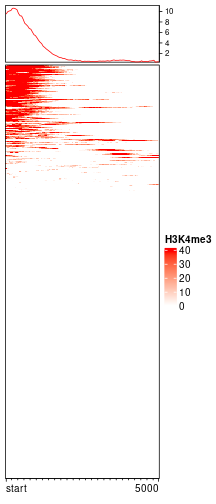### Axis of the enriched annotation Axis of the enriched annotation is controlled by axis_param in anno_enriched(). All the parameters that can be set can be found in ComplexHeatmap::default_axis_param(). EnrichedHeatmap(mat1, col = col_fun, name = "H3K4me3", top_annotation = HeatmapAnnotation( enriched = anno_enriched( ylim = c(0, 10), axis_param = list( at = c(0, 5, 10), labels = c("zero", "five", "ten"), side = "left", facing = "outside" ))) )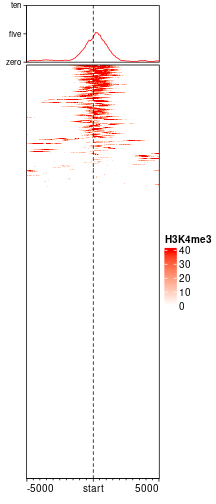### Mean mode When normalizing genomic signals to target regions, upstream and downstream (also target regions themselves if they are included) of the targets are split into small windows. Then genomic signals are overlapped to each window and mean signal for each window is calculated. When a window is not completely covered by the regions for the genomic signales, proper averaging method should be applied to summarize the value in the window. Depending on different scenarios, EnrichedHeatmap provides three metrics for averaging. The overlapping model is illustrated in the following plot. The red line in the bottom represents the small window. Black lines on the top are the regions for genomic signals that overlap with the window. The thick lines indicate the intersected part between the signal regions and the window.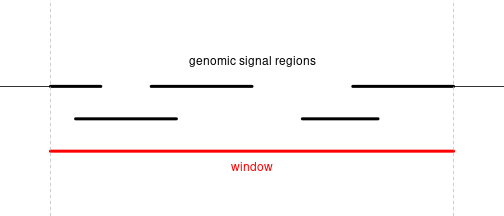For a given window, $$n$$ is the number of signal regions which overlap with the window (it is 5 in the above plot), $$w_i$$ is the width of the intersected segments (black thick lines), and $$x_i$$ is the signal value associated with the original regions. If there is no value associated with the signal regions, $$x_i = 1$$ by default. The “absolute” method is denoted as $$v_a$$ and is simply calculated as the mean of all signal regions regardless of their width: $v_a = \frac{\sum_i^n{x_i}}{n}$ The “weighted” method is denoted as $$v_w$$ and is calculated as the mean of all signal regions weighted by the width of their intersections: $v_w = \frac{\sum_i^n{x_iw_i}}{\sum_i^n{w_i}}$ “Absolute” and “weighted” methods should be applied when background values should not be taken into consideration. For example, when summarizing the mean methylation in a small window, non-CpG background should be ignored, because methylation is only associated with CpG sites and not with other positions. The “w0” method is the weighted mean between the intersected parts and un-intersected parts: $v_{w0} = \frac{v_wW}{W+W'}$ $$W$$ is sum of width of the intersected parts ($$\sum_i^n{w_i}$$) and $$W'$$ is the sum of width for the non-intersected parts. The “coverage” method is denoted as $$v_c$$ and is defined as the mean signal averged by the length of the window: $v_c = \frac{\sum_i^n{x_iw_i}}{L}$ where $$L$$ is the length of the window itself. Note when $$x_i = 1$$, $$v_c$$ is the mean coverage for the signal regions overlapped in the window. Following illustrates different settings for mean_mode (note there is a signal region overlapping with other signal regions):  40 50 20 values in signal regions ++++++ +++ +++++ signal regions 30 values in signal regions ++++++ signal regions ================= window (17bp), there are 4bp not overlapping to any signal region. 4 6 3 3 overlap absolute: (40 + 30 + 50 + 20)/4 weighted: (40*4 + 30*6 + 50*3 + 20*3)/(4 + 6 + 3 + 3) w0: (40*4 + 30*6 + 50*3 + 20*3)/(4 + 6 + 3 + 3 + 4) coverage: (40*4 + 30*6 + 50*3 + 20*3)/17  ### Smoothing Rows can be smoothed by setting smooth to TRUE when generating the matrix. Later we will demonstrate smoothing can also help to impute NA values. As smoothing may change the original data range, the color mapping function col_fun here ensures that the color palette is still the same as the unsmoothed one. background corresponds to the regions that have no signal overlapped. The proper value depends on specific scenarios. Here since we visualize coverage from ChIP-Seq data, it is reasonable to assign 0 to regions with no H3K4me3 signal. In following example, since a enriched heatmap is also a heatmap, we can concatenate two heamtaps by +. mat1_smoothed = normalizeToMatrix(H3K4me3, tss, value_column = "coverage", extend = 5000, mean_mode = "w0", w = 50, background = 0, smooth = TRUE) EnrichedHeatmap(mat1_smoothed, col = col_fun, name = "H3K4me3_smoothed", column_title = "smoothed") + EnrichedHeatmap(mat1, col = col_fun, name = "H3K4me3", column_title = "unsmoothed")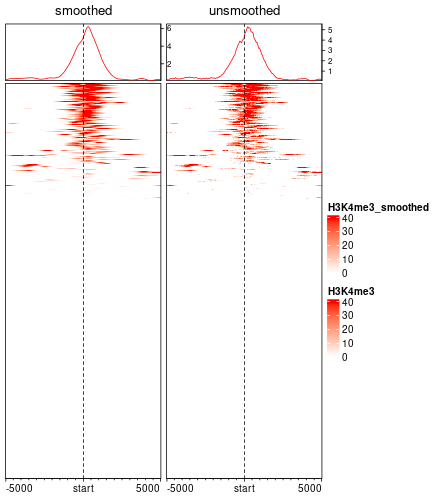In above plots, you might feel the left heatmap is better than the right unsmoothed heatmap. In following, we will demonstrate smoothing can significantly improve the enrichment pattern for methylation datasets. Following heatmap visualizes the enrichment of low methylated regions over TSS. The grey colors represent the windows with no CpG sites (note we set NA to background and grey is the default color for NA values by ComplexHeatmap). meth[1:5]  ## GRanges object with 5 ranges and 1 metadata column: ## seqnames ranges strand | meth ## <Rle> <IRanges> <Rle> | <numeric> ##  chr21 9432427 * | 0.267104 ##  chr21 9432428 * | 0.267107 ##  chr21 9432964 * | 0.272710 ##  chr21 9432965 * | 0.272735 ##  chr21 9433315 * | 0.285115 ## ------- ## seqinfo: 1 sequence from an unspecified genome; no seqlengths  mat2 = normalizeToMatrix(meth, tss, value_column = "meth", mean_mode = "absolute", extend = 5000, w = 50, background = NA) meth_col_fun = colorRamp2(c(0, 0.5, 1), c("blue", "white", "red")) EnrichedHeatmap(mat2, col = meth_col_fun, name = "methylation", column_title = "methylation near TSS")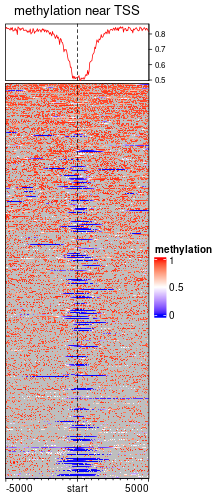When overlapping CpG positions to segmented target regions, it is possible that there is no CpG sites in some windows, especially for meth which only contains 100000 CpG sites which are randomly sampled in chromosome 21. The values for these windows which contain no CpG sites can be imputed by smoothing. Although it seems not proper to assign methylation values to non- CpG windows, but it will improve the visualization a lot. mat2 = normalizeToMatrix(meth, tss, value_column = "meth", mean_mode = "absolute", extend = 5000, w = 50, background = NA, smooth = TRUE) EnrichedHeatmap(mat2, col = meth_col_fun, name = "methylation", column_title = "methylation near TSS")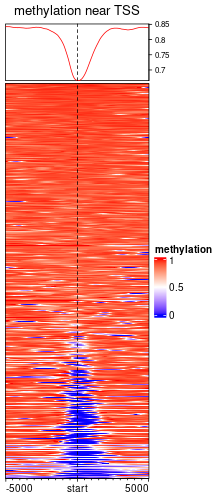To do the smoothing, by default, locfit() is first applied to each row in the original matrix. If it is failed, loess() smoothing is applied afterwards. If both smoothing methods are failed, there will be a warning and the original value is kept. Users can provides their own smoothing function by smooth_fun argument. This self-defined function accepts a numeric vector (may contains NA values) and returns a vector with same length. If the smoothing is failed, the function should call stop() to throw errors so that normalizeToMatrix() can catch how many rows are failed in smoothing. Take a look at the source code of default_smooth_fun() to get an example. Another thing for smoothing methylation data is, methylation is already in a fixed range, i.e. [0, 1], smoothing on raw methylation might produce new values exceeding [0, 1]. Thus, by default, for methylation data (by a guess internally), after smoothing, values will be restricted within [0, 1], as you already saw in the output message in previous example. ### Targets are regions In the example of H3K4me3, the target regions are single points. The targets can also be regions with width larger than 1. Following heatmap visualizes the enrichment of low methylation on CpG islands: mat3 = normalizeToMatrix(meth, cgi, value_column = "meth", mean_mode = "absolute", extend = 5000, w = 50, background = NA, smooth = TRUE, target_ratio = 0.3) EnrichedHeatmap(mat3, col = meth_col_fun, name = "methylation", axis_name_rot = 90, column_title = "methylation near CGI")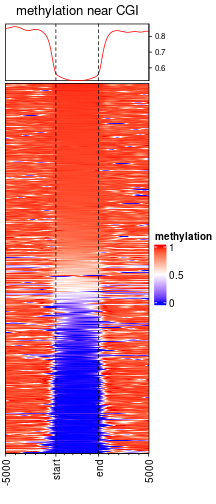Width of the target regions shown on heatmap can be controlled by target_ratio which is relative to the width of the complete heatmap. Target regions are also splitted into small windows. Due to the unequal width of target regions, each target is split into $$k$$ equal windows with $$k = (n_1 +n_2)*r/(1-r)$$ where $$n_1$$ is the number of upstream windows, $$n_2$$ is the number of downstream windows and $$r$$ is the ratio of target columns presented in the matrix. There is a k argument in normalizeToMatrix(), but it is only used when there is no upstream nor downstream for the targets. When genomic targets are regions, upstream and/or downstream can be excluded in the heatmap. mat3 = normalizeToMatrix(meth, cgi, value_column = "meth", mean_mode = "absolute", extend = c(0, 5000), w = 50, background = NA, smooth = TRUE, target_ratio = 0.5) EnrichedHeatmap(mat3, col = meth_col_fun, name = "methylation", column_title = "methylation near CGI")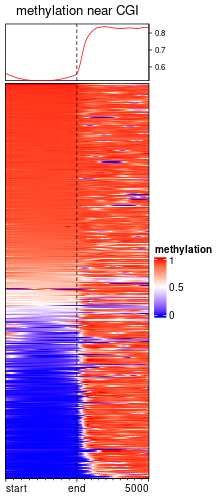mat3 = normalizeToMatrix(meth, cgi, value_column = "meth", mean_mode = "absolute", extend = c(5000, 0), w = 50, background = NA, smooth = TRUE, target_ratio = 0.5)  ## Warning: Smoothing is failed for one row because there are very few signals overlapped to it. ## Please use failed_rows(mat) to get the index of the failed row and consider to remove ## it.  EnrichedHeatmap(mat3, col = meth_col_fun, name = "methylation", column_title = "methylation near CGI")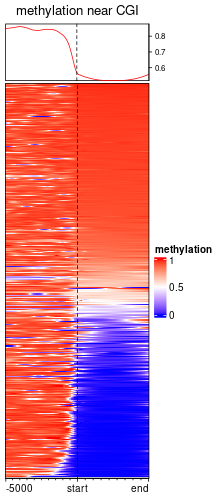When there is no upstream nor downstream, the number of columns in the heatmap is controlled by k argument. mat3 = normalizeToMatrix(meth, cgi, value_column = "meth", mean_mode = "absolute", extend = 0, k = 20, background = NA, smooth = TRUE, target_ratio = 1)  ## Warning: Smoothing is failed for one row because there are very few signals overlapped to it. ## Please use failed_rows(mat) to get the index of the failed row and consider to remove ## it.  EnrichedHeatmap(mat3, col = meth_col_fun, name = "methylation", column_title = "methylation near CGI")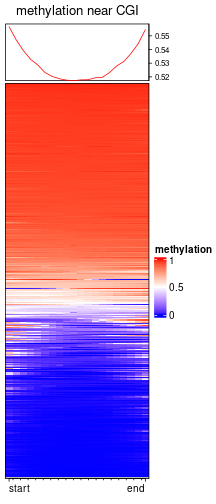You may notice there are warnings when executing above code, that is because there are very few signals overlapped to some rows, which results too many NA values and failed with the smoothing. Corresponding index for failed rows can be get by : failed_rows(mat3)  ##  5  and maybe you can remove this row in the matrix beforehand. ## Multiple heatmaps The power of EnrichedHeatmap package is that parallel heatmaps can be concatenated, both for enriched heatmap, normal heatmap as well the row annotations, which provides a very efficient way to visualize multiple sources of information. With the functionality of ComplexHeatmap package, heatmaps can be concatenated by + operator. Heatmap objects and HeatmapAnnotation objects can be mixed. Following heatmaps visualizes correspondance between H3K4me3 modification, methylation and gene expression. It is quite straightforward to see high expression correlates with low methylation and high H3K4me3 signal around TSS. EnrichedHeatmap(mat1, col = col_fun, name = "H3K4me3", top_annotation = HeatmapAnnotation(enrich = anno_enriched(axis_param = list(side = "left")))) + EnrichedHeatmap(mat2, col = meth_col_fun, name = "methylation") + Heatmap(log2(rpkm+1), col = c("white", "orange"), name = "log2(rpkm+1)", show_row_names = FALSE, width = unit(5, "mm"))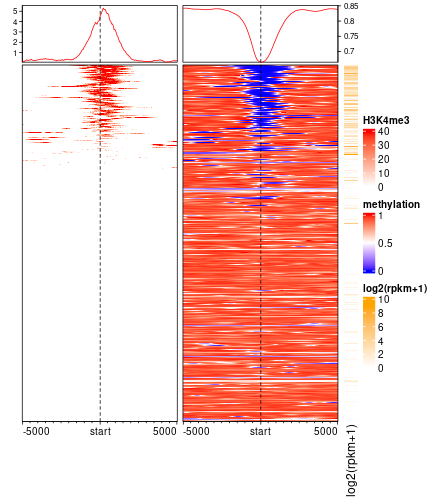Of course you can split rows by partition variables or k-means clustering in the main heatmap. In following heatmaps, the most right color bar can be corresponded to the colors in column annotation on both histone modification heatmap and methylation heatmap. Here we emphasize again, proper trimming on the matrix will greatly help to reveal the patterns. You can try replace mat1 to a un-trimmed matrix and see whether this patterns shown below still preserves. partition = paste0("cluster", kmeans(mat1, centers = 3)$cluster)
lgd = Legend(at = c("cluster1", "cluster2", "cluster3"), title = "Clusters",
type = "lines", legend_gp = gpar(col = 2:4))
ht_list = Heatmap(partition, col = structure(2:4, names = paste0("cluster", 1:3)), name = "partition",
show_row_names = FALSE, width = unit(3, "mm")) +
EnrichedHeatmap(mat1, col = col_fun, name = "H3K4me3",
top_annotation = HeatmapAnnotation(lines = anno_enriched(gp = gpar(col = 2:4))),
column_title = "H3K4me3") +
EnrichedHeatmap(mat2, col = meth_col_fun, name = "methylation",
top_annotation = HeatmapAnnotation(lines = anno_enriched(gp = gpar(col = 2:4))),
column_title = "Methylation") +
Heatmap(log2(rpkm+1), col = c("white", "orange"), name = "log2(rpkm+1)",
show_row_names = FALSE, width = unit(15, "mm"),
top_annotation = HeatmapAnnotation(summary = anno_summary(gp = gpar(fill = 2:4),
outline = FALSE, axis_param = list(side = "right"))))
draw(ht_list, split = partition, annotation_legend_list = list(lgd),
ht_gap = unit(c(2, 8, 8), "mm"))